•神经网络 tensorflow 深度学习
更多相关内容
• 基于改进粒子群算法的深度学习超参数优化方法.pdf
• 基于粒子群优化算法(PSO)的超参数调参

相关文章：

## 1 GlobalBestPSO() 函数简介

PSO 超参数调优采用的是 pyswarm 包中的 GlobalBestPSO()

class pyswarms.single.global_best.GlobalBestPSO(n_particles, dimensions, options, bounds=None, oh_strategy=None, bh_strategy=‘periodic’, velocity_clamp=None, vh_strategy=‘unmodified’, center=1.0, ftol=-inf, ftol_iter=1, init_pos=None)

输入参数：

• n_particles：整数，粒子群中的粒子数量
• dimension：整数，空间维度，或特征维度
• options：系数，字典形式 {‘c1’=2, ‘c2’=2, ‘w’=0.3}
• bounds：数组，可选，行数为 2，第一行是边界的最小值，第二行是边界的最大值
• oh_strategy：字典，可选，每个 option 的选择策略
• bh_strategy：字符串，处理超出边界的粒子的策略
• velocity_clamp：元组，可选，一个大小为2的元组，其中第一个条目是最小速度，第二个条目是最大速度。它设定了速度的限制
• vh_strategy：字符串，处理超出边界的粒子速度的策略
• ftol：列表（默认为None），

GlobalBestPSO()的优化方法

optimize(objective_func, iters, n_processes=None, verbose=True, **kwargs)

输入参数：

• objective_func (function)：自适应函数或损失函数
• iters (int)：迭代次数
• kwargs (dict) ：目标函数的参数

返回：

• tuple：目标函数最优值和最优位置

## 2 定义PSO优化器

import numpy as np
import pyswarms as ps
from sklearn.datasets import make_classification
from sklearn.linear_model import LogisticRegression
from sklearn.metrics import confusion_matrix, classification_report
from sklearn.model_selection import cross_val_score

n_particles = 10
cv = 5
options = {'c1': 0.5, 'c2': 0.5, 'w': 0.9}
optimizer = ps.single.GlobalBestPSO(n_particles=n_particles, dimensions=dims,
options=options, bounds=bounds)


## 3 定义参数和边界

这里选择逻辑回归模型的超参数：C 和 penalty。

# 超参数 C 和 penalty
C = [0., 1.]
penalty = ['l1', 'l2', 'elasticnet', 'none']
penalty_bound = [0, len(penalty)]  # 把这个看作索引
dims = 2

# bounds表示所有参数的界限
max_ = np.zeros(dims)
min_ = np.zeros(dims)

for i, param in enumerate([C, penalty_bound]):
min_[i] = param
max_[i] = param

bounds = (min_, max_)
print(bounds)


输出：

(array([0., 0.]), array([1., 4.]))


## 4 得到最优模型

# 这里目标函数的输入为需要优化的参数
best_cost, best_pos = optimizer.optimize(objective, iters=25)
print(best_pos, penalty[int(best_pos)])
best_model = LogisticRegression(C=best_pos, penalty=penalty[int(best_pos)])
best_model = best_model.fit(X_train, y_train)


输出：

[0.01806252 1.430832  ] l2


## 5 定义目标函数

在定义目标函数时，需要将每个粒子的值带入模型中训练，然后返回模型的指标值。这个指标值可以是分类中的准确率。

具体如下：

def objective(param):
"""计算每个粒子的适应度，param表示输入的参数(n_particles, n_dims)"""
scores = np.zeros((n_particles, cv))
clf = LogisticRegression
for i in range(n_particles):
"""计算每个粒子的得分"""
scores[i, :] = cross_val_score(clf(C=param[i], penalty=penalty[int(param[i])]),
X_train, y_train,
scoring='accuracy',
cv=cv)
return -np.mean(scores, axis=1)


## 6 查看损失变化

查看目标函数随迭代增加的变化情况：

from pyswarms.utils.plotters import plot_cost_history
plot_cost_history(cost_history=optimizer.cost_history)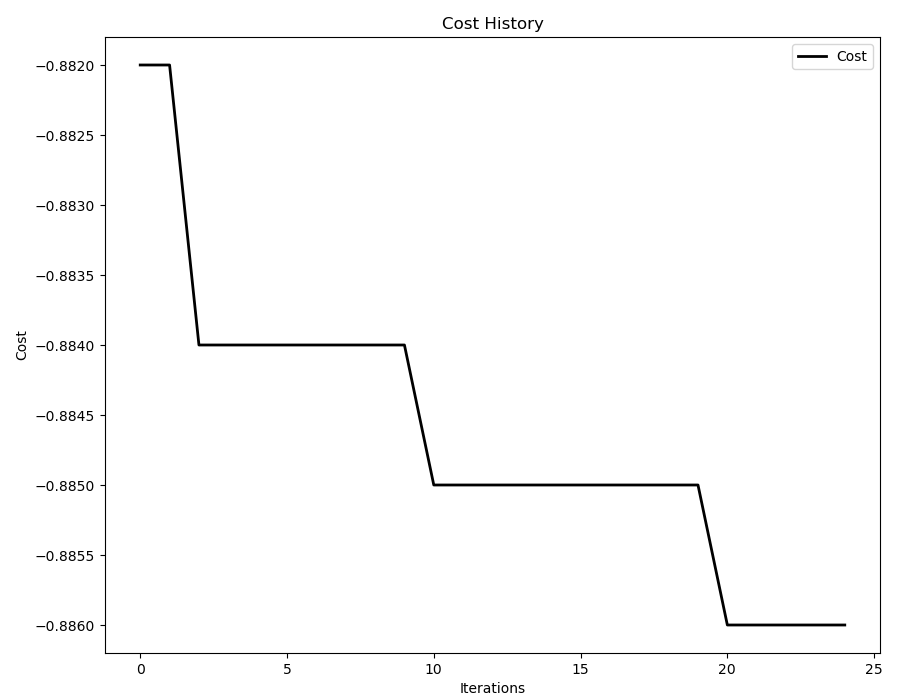## 7 测试模型

X, y = make_classification(n_samples=2000, n_features=5, random_state=42)

X_train, y_train = X[:1000], y[:1000]
X_test, y_test = X[1000:], y[1000:]

y_pred = best_model.predict(X_test)
print(confusion_matrix(y_test, y_pred))
print(classification_report(y_test, y_pred))


输出：

[[440  55]
[ 45 460]]
precision    recall  f1-score   support
0       0.91      0.89      0.90       495
1       0.89      0.91      0.90       505
accuracy                           0.90      1000
macro avg       0.90      0.90      0.90      1000
weighted avg       0.90      0.90      0.90      1000

展开全文分类 数据挖掘 python
• 【寻优算法】粒子群算法（PSO） 参数寻优的python实现一、算法原理1、粒子群算法的名词解释2、粒子更新二、PSO算法参数寻优的python实现参考资料 粒子群优化算法（Particle swarm optimization，PSO）是模拟鸟群...

### 【超参数寻优】粒子群算法（PSO） 超参数寻优的python实现

粒子群优化算法（Particle swarm optimization，PSO）是模拟鸟群捕食行为的优化算法。不同于遗传算法（Genetic Alogrithm，GA），粒子群算法是有记忆的，之前迭代过程中的最优位置和最优方向都会保留下来并作用于粒子群的更新【参考资料1】。

# 一、算法原理

## 1、粒子群算法的名词解释

粒子群长度：粒子群长度等于每一个参数取值范围的大小。
粒子群维度：粒子群维度等于待寻优参数的数量。
粒子群位置：粒子群位置包含参数取值的具体数值。
粒子群方向：粒子群方向表示参数取值的变化方向。
适应度函数：表征粒子对应的模型评价指标。
pbest:（局部最优）pbest的长度等于粒子群长度，表示每一个参数取值的变化过程中，到目前为止最优适应度函数值对应的取值。
gbest:（全局最优）gbest的长度为1，表示到目前为止所有适应度函数值中最优的那个对应的参数取值。
惯性因子 w w ：惯性因子表示粒子保持的运动惯性。
局部学习因子 c 1 {c_1} ：表示每个粒子向该粒子目前为止最优位置运动加速项的权重。
全局学习因子 c 2 {c_2} ：表示每个粒子向目前为止全局最优位置运动加速项的权重。

## 2、粒子更新

粒子方向更新方程为：

v i = w × v i + c 1 × r a n d ( ) × ( p b e s t i − x i ) + c 2 × r a n d ( ) × ( g b e s t − x i ) {v_i} = w \times {v_i} + {c_1} \times rand() \times (pbes{t_i} - {x_i}) + {c_2} \times rand() \times (gbest - {x_i})

其中 v i {v_i} 表示第i个粒子的方向， p b e s t i pbes{t_i} 表示第i个粒子当前最优参数， g b e s t gbest 表示当前的全局最优参数， x i x_i 表示第i个粒子的参数值， r a n d ( ) rand() 表示介于 ( 0 , 1 ) (0,1) 之间的随机数。

粒子位置更新方程为：

x i = x i + v i {x_i} = {x_i} + {v_i}

w w 取值越大说明粒子更新更多受到粒子历史方向的影响， w = 0 w=0 表示粒子更新只取决于粒子当前位置，局部最优粒子位置和全局最优粒子位置，粒子方向本身没有记忆性。 c 1 {c_1} 表示粒子更新受到局部最优粒子的影响程度， c 1 = 0 {c_1}=0 表示粒子只有全局搜索能力，但缺少局部搜索能力。 c 2 {c_2} 表示粒子更新受到全局最优粒子的影响程度， c 2 = 0 {c_2}=0 表示粒子只有局部搜索能力，但缺少全局搜索能力。

# 二、PSO算法参数寻优的python实现

完整python代码和样本地址：https://github.com/shiluqiang/PSO_python

本博文以非线性SVM为待优化模型，待优化参数为正则化参数 C C 和核参数 σ \sigma ，适应度函数值为3-fold交叉验证平均值。

## 2. PSO优化算法
class PSO(object):
def __init__(self,particle_num,particle_dim,iter_num,c1,c2,w,max_value,min_value):
'''参数初始化
particle_num(int):粒子群的粒子数量
particle_dim(int):粒子维度，对应待寻优参数的个数
iter_num(int):最大迭代次数
c1(float):局部学习因子，表示粒子移动到该粒子历史最优位置(pbest)的加速项的权重
c2(float):全局学习因子，表示粒子移动到所有粒子最优位置(gbest)的加速项的权重
w(float):惯性因子，表示粒子之前运动方向在本次方向上的惯性
max_value(float):参数的最大值
min_value(float):参数的最小值
'''
self.particle_num = particle_num
self.particle_dim = particle_dim
self.iter_num = iter_num
self.c1 = c1  ##通常设为2.0
self.c2 = c2  ##通常设为2.0
self.w = w
self.max_value = max_value
self.min_value = min_value

### 2.1 粒子群初始化
def swarm_origin(self):
'''粒子群初始化
input:self(object):PSO类
output:particle_loc(list):粒子群位置列表
particle_dir(list):粒子群方向列表
'''
particle_loc = []
particle_dir = []
for i in range(self.particle_num):
tmp1 = []
tmp2 = []
for j in range(self.particle_dim):
a = random.random()
b = random.random()
tmp1.append(a * (self.max_value - self.min_value) + self.min_value)
tmp2.append(b)
particle_loc.append(tmp1)
particle_dir.append(tmp2)

return particle_loc,particle_dir

## 2.2 计算适应度函数数值列表;初始化pbest_parameters和gbest_parameter
def fitness(self,particle_loc):
'''计算适应度函数值
input:self(object):PSO类
particle_loc(list):粒子群位置列表
output:fitness_value(list):适应度函数值列表
'''
fitness_value = []
### 1.适应度函数为RBF_SVM的3_fold交叉校验平均值
for i in range(self.particle_num):
rbf_svm = svm.SVC(kernel = 'rbf', C = particle_loc[i], gamma = particle_loc[i])
cv_scores = cross_validation.cross_val_score(rbf_svm,trainX,trainY,cv =3,scoring = 'accuracy')
fitness_value.append(cv_scores.mean())
### 2. 当前粒子群最优适应度函数值和对应的参数
current_fitness = 0.0
current_parameter = []
for i in range(self.particle_num):
if current_fitness < fitness_value[i]:
current_fitness = fitness_value[i]
current_parameter = particle_loc[i]

return fitness_value,current_fitness,current_parameter

## 2.3  粒子位置更新
def updata(self,particle_loc,particle_dir,gbest_parameter,pbest_parameters):
'''粒子群位置更新
input:self(object):PSO类
particle_loc(list):粒子群位置列表
particle_dir(list):粒子群方向列表
gbest_parameter(list):全局最优参数
pbest_parameters(list):每个粒子的历史最优值
output:particle_loc(list):新的粒子群位置列表
particle_dir(list):新的粒子群方向列表
'''
## 1.计算新的量子群方向和粒子群位置
for i in range(self.particle_num):
a1 = [x * self.w for x in particle_dir[i]]
a2 = [y * self.c1 * random.random() for y in list(np.array(pbest_parameters[i]) - np.array(particle_loc[i]))]
a3 = [z * self.c2 * random.random() for z in list(np.array(gbest_parameter) - np.array(particle_dir[i]))]
particle_dir[i] = list(np.array(a1) + np.array(a2) + np.array(a3))
#            particle_dir[i] = self.w * particle_dir[i] + self.c1 * random.random() * (pbest_parameters[i] - particle_loc[i]) + self.c2 * random.random() * (gbest_parameter - particle_dir[i])
particle_loc[i] = list(np.array(particle_loc[i]) + np.array(particle_dir[i]))

## 2.将更新后的量子位置参数固定在[min_value,max_value]内
### 2.1 每个参数的取值列表
parameter_list = []
for i in range(self.particle_dim):
tmp1 = []
for j in range(self.particle_num):
tmp1.append(particle_loc[j][i])
parameter_list.append(tmp1)
### 2.2 每个参数取值的最大值、最小值、平均值
value = []
for i in range(self.particle_dim):
tmp2 = []
tmp2.append(max(parameter_list[i]))
tmp2.append(min(parameter_list[i]))
value.append(tmp2)

for i in range(self.particle_num):
for j in range(self.particle_dim):
particle_loc[i][j] = (particle_loc[i][j] - value[j])/(value[j] - value[j]) * (self.max_value - self.min_value) + self.min_value

return particle_loc,particle_dir

## 2.4 画出适应度函数值变化图
def plot(self,results):
'''画图
'''
X = []
Y = []
for i in range(self.iter_num):
X.append(i + 1)
Y.append(results[i])
plt.plot(X,Y)
plt.xlabel('Number of iteration',size = 15)
plt.ylabel('Value of CV',size = 15)
plt.title('PSO_RBF_SVM parameter optimization')
plt.show()

## 2.5 主函数
def main(self):
'''主函数
'''
results = []
best_fitness = 0.0
## 1、粒子群初始化
particle_loc,particle_dir = self.swarm_origin()
## 2、初始化gbest_parameter、pbest_parameters、fitness_value列表
### 2.1 gbest_parameter
gbest_parameter = []
for i in range(self.particle_dim):
gbest_parameter.append(0.0)
### 2.2 pbest_parameters
pbest_parameters = []
for i in range(self.particle_num):
tmp1 = []
for j in range(self.particle_dim):
tmp1.append(0.0)
pbest_parameters.append(tmp1)
### 2.3 fitness_value
fitness_value = []
for i in range(self.particle_num):
fitness_value.append(0.0)

## 3.迭代
for i in range(self.iter_num):
### 3.1 计算当前适应度函数值列表
current_fitness_value,current_best_fitness,current_best_parameter = self.fitness(particle_loc)
### 3.2 求当前的gbest_parameter、pbest_parameters和best_fitness
for j in range(self.particle_num):
if current_fitness_value[j] > fitness_value[j]:
pbest_parameters[j] = particle_loc[j]
if current_best_fitness > best_fitness:
best_fitness = current_best_fitness
gbest_parameter = current_best_parameter

print('iteration is :',i+1,';Best parameters:',gbest_parameter,';Best fitness',best_fitness)
results.append(best_fitness)
### 3.3 更新fitness_value
fitness_value = current_fitness_value
### 3.4 更新粒子群
particle_loc,particle_dir = self.updata(particle_loc,particle_dir,gbest_parameter,pbest_parameters)
## 4.结果展示
results.sort()
self.plot(results)
print('Final parameters are :',gbest_parameter)


最优适应度函数值随迭代次数的变化图如下：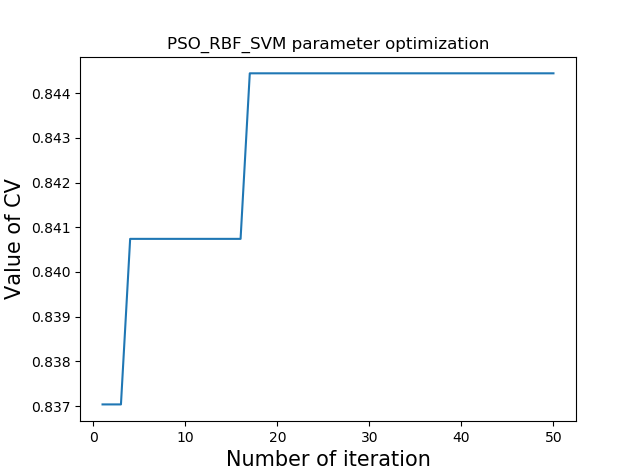# 参考资料

展开全文python
• 一种基于改进粒子群算法的PID参数整定方法，使用粒子群算法对PID参数优化
• 基于遗传算法优化多尺度排列熵参数，类似于粒子群算法优化参数
• ## 最优化算法之粒子群算法（PSO）

万次阅读 多人点赞 2018-08-03 10:26:45
一、粒子群算法的概念   粒子群优化算法(PSO：Particle swarm optimization) 是一种进化计算技术（evolutionary computation）。源于对鸟群捕食的行为研究。粒子群优化算法的基本思想：是通过群体中个体之间的...

一、粒子群算法的概念

粒子群优化算法(PSO：Particle swarm optimization) 是一种进化计算技术（evolutionary computation）。源于对鸟群捕食的行为研究。粒子群优化算法的基本思想：是通过群体中个体之间的协作和信息共享来寻找最优解．
PSO的优势：在于简单容易实现并且没有许多参数的调节。目前已被广泛应用于函数优化、神经网络训练、模糊系统控制以及其他遗传算法的应用领域。

二、粒子群算法分析

1、基本思想

粒子群算法通过设计一种无质量的粒子来模拟鸟群中的鸟，粒子仅具有两个属性：速度和位置，速度代表移动的快慢，位置代表移动的方向。每个粒子在搜索空间中单独的搜寻最优解，并将其记为当前个体极值，并将个体极值与整个粒子群里的其他粒子共享，找到最优的那个个体极值作为整个粒子群的当前全局最优解，粒子群中的所有粒子根据自己找到的当前个体极值和整个粒子群共享的当前全局最优解来调整自己的速度和位置。下面的动图很形象地展示了PSO算法的过程：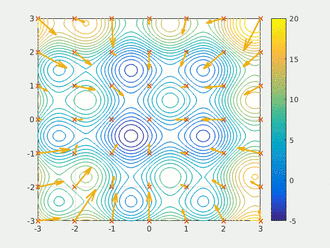2、更新规则

PSO初始化为一群随机粒子(随机解)。然后通过迭代找到最优解。在每一次的迭代中，粒子通过跟踪两个“极值”(pbest，gbest)来更新自己。在找到这两个最优值后，粒子通过下面的公式来更新自己的速度和位置。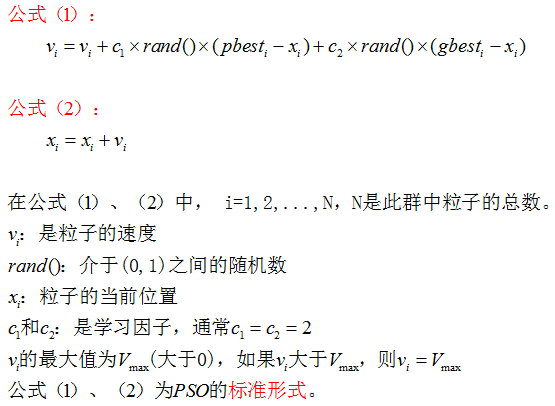公式(1)的第一部分称为【记忆项】，表示上次速度大小和方向的影响；公式(1)的第二部分称为【自身认知项】，是从当前点指向粒子自身最好点的一个矢量，表示粒子的动作来源于自己经验的部分；公式(1)的第三部分称为【群体认知项】，是一个从当前点指向种群最好点的矢量，反映了粒子间的协同合作和知识共享。粒子就是通过自己的经验和同伴中最好的经验来决定下一步的运动。以上面两个公式为基础，形成了PSO的标准形式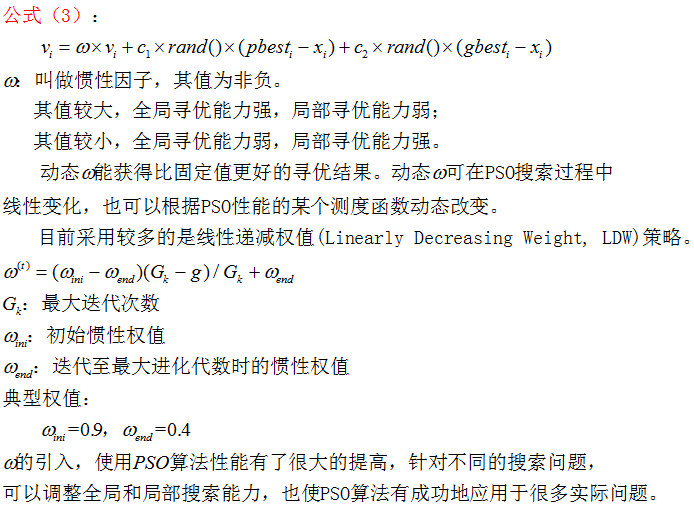公式(2)和 公式(3)被视为标准PSO算法

3、PSO算法的流程和伪代码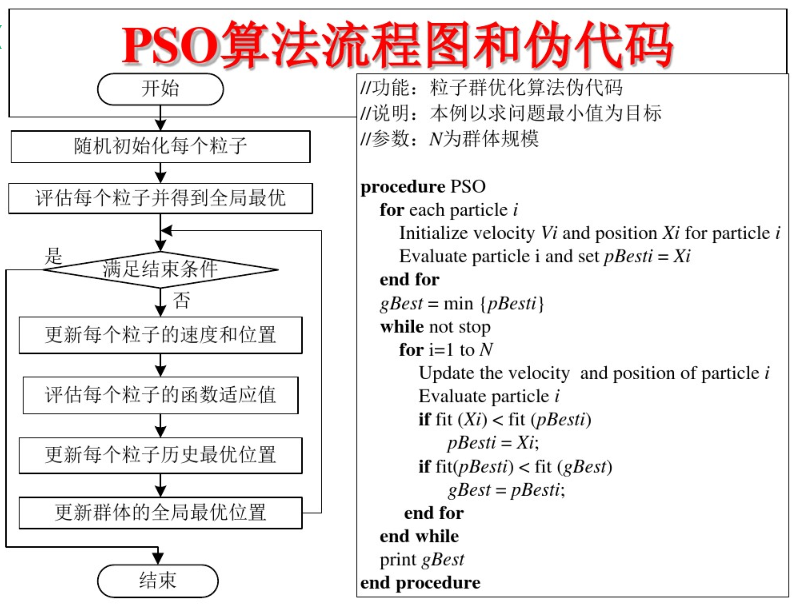4、PSO算法举例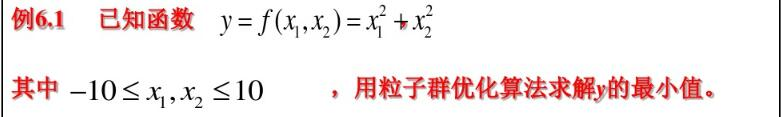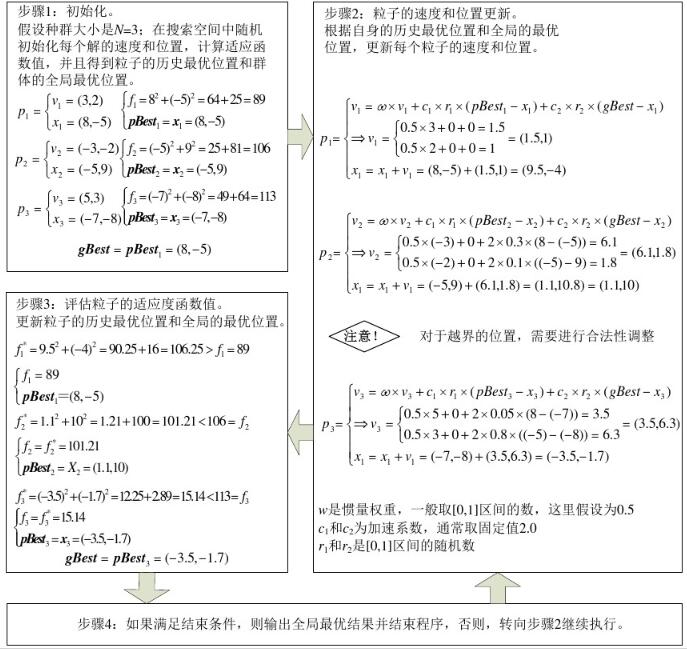5、PSO算法的demo

#include <iostream>
#include <vector>
#include <cmath>
#include <map>
#include <algorithm>
#include <random>
#include <ctime>
#include <Eigen/Dense>
using namespace Eigen;
using namespace std;

const int dim = 1;//维数
const int p_num = 10;//粒子数量
const int iters = 100;//迭代次数
const int inf = 999999;//极大值
const double pi = 3.1415;
//定义粒子的位置和速度的范围
const double v_max = 4;
const double v_min = -2;
const double pos_max = 2;
const double pos_min = -1;
//定义位置向量和速度向量
vector<double> pos;
vector<double> spd;
//定义粒子的历史最优位置和全局最优位置
vector<double> p_best;
double g_best;
//使用eigen库定义函数值矩阵和位置矩阵
Matrix<double, iters, p_num> f_test;
Matrix<double, iters, p_num> pos_mat;

//定义适应度函数
double fun_test(double x)
{
double res = x * x + 1;
return res;
}

//初始化粒子群的位置和速度
void init()
{
//矩阵中所有元素初始化为极大值
f_test.fill(inf);
pos_mat.fill(inf);
//生成范围随机数
static std::mt19937 rng;
static std::uniform_real_distribution<double> distribution1(-1, 2);
static std::uniform_real_distribution<double> distribution2(-2, 4);
for (int i = 0; i < p_num; ++i)
{
pos.push_back(distribution1(rng));
spd.push_back(distribution2(rng));
}
vector<double> vec;
for (int i = 0; i < p_num; ++i)
{
auto temp = fun_test(pos[i]);//计算函数值
//初始化函数值矩阵和位置矩阵
f_test(0, i) = temp;
pos_mat(0, i) = pos[i];
p_best.push_back(pos[i]);//初始化粒子的历史最优位置
}
std::ptrdiff_t minRow, minCol;
f_test.row(0).minCoeff(&minRow, &minCol);//返回函数值矩阵第一行中极小值对应的位置
g_best = pos_mat(minRow, minCol);//初始化全局最优位置
}

void PSO()
{
static std::mt19937 rng;
static std::uniform_real_distribution<double> distribution(0, 1);
for (int step = 1; step < iters; ++step)
{
for (int i = 0; i < p_num; ++i)
{
//更新速度向量和位置向量
spd[i] = 0.5 * spd[i] + 2 * distribution(rng) * (p_best[i] - pos[i]) +
2 * distribution(rng) * (g_best - pos[i]);
pos[i] = pos[i] + spd[i];
//如果越界则取边界值
if (spd[i] < -2 || spd[i] > 4)
spd[i] = 4;
if (pos[i] < -1 || pos[i] > 2)
pos[i] = -1;
//更新位置矩阵
pos_mat(step, i) = pos[i];
}
//更新函数值矩阵
for (int i = 0; i < p_num; ++i)
{
auto temp = fun_test(pos[i]);
f_test(step, i) = temp;
}
for (int i = 0; i < p_num; ++i)
{
MatrixXd temp_test;
temp_test = f_test.col(i);//取函数值矩阵的每一列
std::ptrdiff_t minRow, minCol;
temp_test.minCoeff(&minRow, &minCol);//获取每一列的极小值对应的位置
p_best[i] = pos_mat(minRow, i);//获取每一列的极小值，即每个粒子的历史最优位置
}
g_best = *min_element(p_best.begin(), p_best.end());//获取全局最优位置
}
cout << fun_test(g_best);
}

int main()
{
init();
PSO();
system("pause");
return 0;
}
展开全文• 高斯过程回归超参数自适应选择粒子群优化算法.pdf
• 因此,采用粒子群优化(Particle Swarm Optimization,PSO)算法对LS-SVM算法寻找最优超参数,进一步提高LS-SVM对电动机断条故障诊断的效率和准确率有着重要作用。实验结果表明,综合PSO与LS-SVM两种算法的优点,可有效减少...
• 此函数适合给定模型的计算参数，该参数最适合给定的实验测量值，并受非线性等式 -funCEq 和不等式约束 -funCInEq 的约束，并且在 lb 和 ub 之间的框内matlab
• 超参数寻优】量子粒子群算法（QPSO） 超参数寻优的python实现 一、粒子群算法的缺点 二、量子粒子群算法 三、QPSO算法的python实现 参考资料 一、粒子群算法的缺点 本人之前的博文（参考资料【1】）已经...
• 粒子群优化算法对BPNN进行超参数优化的python代码实现，亲测可用
• 粒子群优化算法（Particle Swarm Optimization，PSO）简介。
• 粒子群优化SVM 其中代码部分经过测试，实测可用 步骤讲解 1、粒子群优化的SVM的c和g,由于SVM中的c和g难以选择最优的，故选择PSO来优化，寻找最优的粒子点来作为SVM的c和g。 2、从随机解出发，通过迭代寻找最优解，...机器学习
• 粒子群算法的构成要素分析 C++程序应用实例1 C++程序应用实例2 总结 visual studio2017c++源代码 源文件下载地址 个人申明 基本概念 粒子群优化算法（particle swarm optimization，PSO）属于进化算法...源代码双闭环直流调速 PID
• 针对四旋翼飞行器自抗扰控制器参数较多、人工整定困难且难以得到最优控制效果的问题，提出一种基于改进粒子群算法的四旋翼自抗扰控制器优化方法。在设计了四旋翼飞行器的自抗扰控制器之后，将自抗扰控制器的参数作为...四旋翼飞行器
• 一、粒子群算法优化支持向量机简介 1 SVM SVM是监督学习中最有影响力的方法之一。其基本模型是定义在空间上最大间隔的线性分类器，由于其遵循经验风险与置信风险之和最小化（即结构风险）原理，因此SVM泛化能力强。...支持向量机 matlab
• 粒子群优化算法(PSO：Particle swarm optimization) 是一种进化计算技术（evolutionary computation）。源于对鸟群捕食的行为研究。粒子群优化算法的基本思想：是通过群体中个体之间的协作和信息共享来寻找最优解． ...python 线性回归 github
• 这里提供一种加快反向传播的算法，目的是在训练神经网络时不使用反向传播以及梯度下降算法，而是先使用粒子群优化算法（Particle Swarm Optimization，PSO）对网络参数进行初始化，之后可以再次使用反向传播对网络...深度学习 神经网络
• 一、粒子群算法及RBF简介 1 粒子群算法简介 1.1 引言 自然界中的鸟群和鱼群的群体行为一直是科学家的研究兴趣所在。生物学家Craig Reynolds在1987年提出了一个非常有影响的鸟群聚集模型，在他的仿真中，每一个个体都...matlab 神经网络
• 粒子群优化，又称微粒群算法，来源于对—个简化社会模型的模拟，主要用于求解优化问题。 粒子群优化算法是 Kennedy和 Eberhart受人工生命硏究结果的启发，通过模拟鸟群觅食过程中的迁徙和群聚行为而提出的一种...
• 摘要：整理了相关改进粒子群算法的代码，方便大家学习,大家可以借鉴相应的思想去优化其他算法。 代码内容： 1.基于粒子群算法的路径规划（PSO Path Planning）。 2.二进制粒子群算法（BinaryPSO） Khanesar, M.A., ...人工智能
• 粒子群算法求解带约束优化问题 源码实现 python实现。带你体验下粒子群算法是如何求解带等式约束、不等式约束、上下限约束的问题。python
• 首先对病灶图片进行gabor小波纹理特征提取，然后输入进经粒子群优化的支持向量机进行训练与测试分类​。 支持向量机 (Support Vector Machines, SVM) 是一种应用广泛的机器学习方法, 具有理论知识清晰完备,适应性和...分类 支持向量机
• ## PSO粒子群算法调节PID控制器参数

千次阅读 多人点赞 2020-03-09 19:05:41
PSO（粒子群算法）在处理连续问题上有着较强的能力，因此很适合用来做参数优化，而PID控制器由三个参数组成，它们分别是： Kp、Ki、KdK_p、K_i、K_dKp​、Ki​、Kd​ 我们可以把PID控制器当做一个“黑箱”，输入为这...矩阵 pid
• 粒子群算法，也称粒子群优化算法或鸟群觅食算法（Particle Swarm Optimization），缩写为 PSO， 是近年来由J. Kennedy和R. C. Eberhart等开发的一种新的进化算法(Evolutionary Algorithm - EA)。PSO 算法属于进化...matlab
• 支持向量机 (Support Vector Machines, SVM) 是一种应用广泛的机器学习方法, 具有理论知识清晰完备,适应性和泛化能力良好的优点, 核心思想是在特征空间中寻找到一个最优平面将两类样本尽可能大的分开, 能够较好的...matlab 支持向量机...